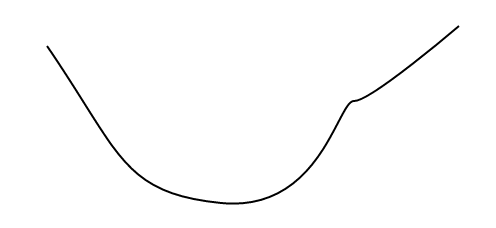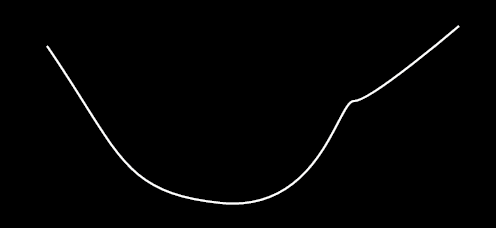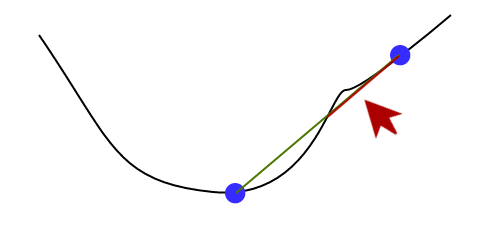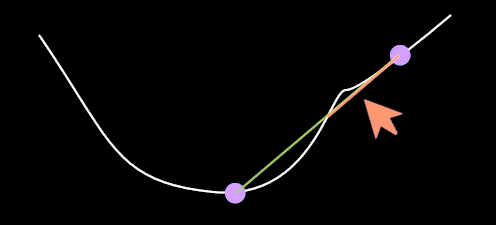# Convex function

A convex function “only curves upwards.” To check whether a function is convex, use the following procedure:

1. Draw the graph of the function.1. Select any two points in the graph.1. Draw a line segment connecting the two points.1. See whether this line segment is ever lower than the graph.If the line segment is ever lower than the graph, the function is not convex. The function graphed above is not convex, as can be seen by looking at the red part of the line segment. On the other hand, if the line segment never goes below the graph (no matter which two initial points you selected), then the function is convex.

Equivalently, a function is convex if its epigraph is a convex set.

Parents: